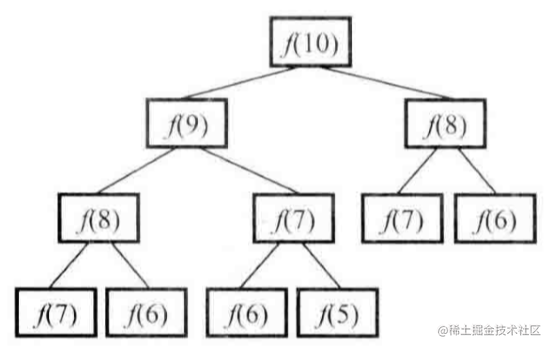# 剑指 Offer 每日一题 | 7、斐波那契数列### 二、题目

 F(0) = 0,   F(1) = 1
F(N) = F(N - 1) + F(N - 2), 其中 N > 1.

 输入：n = 2
输出：1

 输入：n = 5
输出：5

• 0<=n<=100

### 三、解题

#### 3.1 思路1：递归##### 3.1.1 代码
    // 1、递归
class Solution{
public int f(int n){
if(n>1){
return f(n-1)+f(n-2);
}else if(n==1){
return 1;
}else{
return 0;
}
}
}

##### 3.1.2 运行效果#### 3.2 思路2：动态规划

• 1、找到“状态“和“选择”
• 2、明确dp数组函数定义
• 3、寻找状态之间的关系

n01234567
f(n)011235813

##### 3.2.1 代码
     // 2、动态规划
static class Solution2{
public int f(int n){
if(n>1){
// 原地动态规划，不占用额外空间 f(n+1)=f(n)+f(n-1)
int left=0;
int right =1;
for (int i = 0; i <n; i++) {
int tmp =(left+right)%1000000007;
left=right;
right=tmp;
}
return left;
}else if(n==1){
return 1;
}else{
return 0;
}
}
}

##### 3.2.2 执行效果##### 3.2.3 复杂度
• 时间复杂度O（n）：计算f（n）需要循环n次
• 空间复杂度O（1）：几个变量使用额外空间O（1）